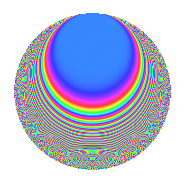# Properties

 Label 735.2.aLevel 735 Weight 2 Character orbit a Rep. character $$\chi_{735}(1,\cdot)$$ Character field $$\Q$$ Dimension 28 Newform subspaces 15 Sturm bound 224 Trace bound 4

# Related objects

## Defining parameters

 Level: $$N$$ = $$735 = 3 \cdot 5 \cdot 7^{2}$$ Weight: $$k$$ = $$2$$ Character orbit: $$[\chi]$$ = 735.a (trivial) Character field: $$\Q$$ Newform subspaces: $$15$$ Sturm bound: $$224$$ Trace bound: $$4$$ Distinguishing $$T_p$$: $$2$$, $$13$$

## Dimensions

The following table gives the dimensions of various subspaces of $$M_{2}(\Gamma_0(735))$$.

Total New Old
Modular forms 128 28 100
Cusp forms 97 28 69
Eisenstein series 31 0 31

The following table gives the dimensions of the cuspidal new subspaces with specified eigenvalues for the Atkin-Lehner operators and the Fricke involution.

$$3$$$$5$$$$7$$FrickeDim.
$$+$$$$+$$$$+$$$$+$$$$2$$
$$+$$$$+$$$$-$$$$-$$$$4$$
$$+$$$$-$$$$+$$$$-$$$$6$$
$$+$$$$-$$$$-$$$$+$$$$1$$
$$-$$$$+$$$$+$$$$-$$$$5$$
$$-$$$$+$$$$-$$$$+$$$$3$$
$$-$$$$-$$$$+$$$$+$$$$1$$
$$-$$$$-$$$$-$$$$-$$$$6$$
Plus space$$+$$$$7$$
Minus space$$-$$$$21$$

## Trace form

 $$28q + 4q^{2} + 2q^{3} + 32q^{4} - 2q^{6} + 12q^{8} + 28q^{9} + O(q^{10})$$ $$28q + 4q^{2} + 2q^{3} + 32q^{4} - 2q^{6} + 12q^{8} + 28q^{9} + 8q^{11} + 6q^{12} + 8q^{13} - 2q^{15} + 48q^{16} + 4q^{18} + 8q^{20} + 24q^{22} - 8q^{23} + 6q^{24} + 28q^{25} + 24q^{26} + 2q^{27} + 24q^{29} - 2q^{30} - 16q^{31} + 28q^{32} + 32q^{36} - 4q^{37} - 8q^{38} - 24q^{39} - 20q^{43} + 8q^{44} + 8q^{46} - 24q^{47} - 2q^{48} + 4q^{50} + 4q^{51} - 8q^{52} + 32q^{53} - 2q^{54} + 8q^{55} - 12q^{57} + 16q^{58} - 6q^{60} + 8q^{61} - 24q^{62} + 72q^{64} + 8q^{65} - 16q^{66} - 20q^{67} + 16q^{68} + 16q^{71} + 12q^{72} + 8q^{73} - 32q^{74} + 2q^{75} - 16q^{76} - 4q^{78} - 28q^{79} + 28q^{81} + 16q^{82} + 8q^{83} - 8q^{85} - 112q^{86} - 4q^{87} - 112q^{88} + 16q^{89} - 160q^{92} - 20q^{93} + 40q^{94} + 16q^{95} - 10q^{96} + 8q^{97} + 8q^{99} + O(q^{100})$$

## Decomposition of $$S_{2}^{\mathrm{new}}(\Gamma_0(735))$$ into newform subspaces

Label Dim. $$A$$ Field CM Traces A-L signs $q$-expansion
$$a_2$$ $$a_3$$ $$a_5$$ $$a_7$$ 3 5 7
735.2.a.a $$1$$ $$5.869$$ $$\Q$$ None $$-2$$ $$-1$$ $$-1$$ $$0$$ $$+$$ $$+$$ $$-$$ $$q-2q^{2}-q^{3}+2q^{4}-q^{5}+2q^{6}+q^{9}+\cdots$$
735.2.a.b $$1$$ $$5.869$$ $$\Q$$ None $$-2$$ $$1$$ $$1$$ $$0$$ $$-$$ $$-$$ $$+$$ $$q-2q^{2}+q^{3}+2q^{4}+q^{5}-2q^{6}+q^{9}+\cdots$$
735.2.a.c $$1$$ $$5.869$$ $$\Q$$ None $$-1$$ $$1$$ $$-1$$ $$0$$ $$-$$ $$+$$ $$-$$ $$q-q^{2}+q^{3}-q^{4}-q^{5}-q^{6}+3q^{8}+\cdots$$
735.2.a.d $$1$$ $$5.869$$ $$\Q$$ None $$0$$ $$-1$$ $$1$$ $$0$$ $$+$$ $$-$$ $$-$$ $$q-q^{3}-2q^{4}+q^{5}+q^{9}+2q^{12}+q^{13}+\cdots$$
735.2.a.e $$1$$ $$5.869$$ $$\Q$$ None $$0$$ $$1$$ $$-1$$ $$0$$ $$-$$ $$+$$ $$+$$ $$q+q^{3}-2q^{4}-q^{5}+q^{9}-2q^{12}-q^{13}+\cdots$$
735.2.a.f $$1$$ $$5.869$$ $$\Q$$ None $$1$$ $$-1$$ $$-1$$ $$0$$ $$+$$ $$+$$ $$-$$ $$q+q^{2}-q^{3}-q^{4}-q^{5}-q^{6}-3q^{8}+\cdots$$
735.2.a.g $$2$$ $$5.869$$ $$\Q(\sqrt{3})$$ None $$-2$$ $$-2$$ $$2$$ $$0$$ $$+$$ $$-$$ $$+$$ $$q+(-1+\beta )q^{2}-q^{3}+(2-2\beta )q^{4}+q^{5}+\cdots$$
735.2.a.h $$2$$ $$5.869$$ $$\Q(\sqrt{3})$$ None $$-2$$ $$2$$ $$-2$$ $$0$$ $$-$$ $$+$$ $$-$$ $$q+(-1+\beta )q^{2}+q^{3}+(2-2\beta )q^{4}-q^{5}+\cdots$$
735.2.a.i $$2$$ $$5.869$$ $$\Q(\sqrt{2})$$ None $$0$$ $$-2$$ $$-2$$ $$0$$ $$+$$ $$+$$ $$+$$ $$q+\beta q^{2}-q^{3}-q^{5}-\beta q^{6}-2\beta q^{8}+\cdots$$
735.2.a.j $$2$$ $$5.869$$ $$\Q(\sqrt{2})$$ None $$0$$ $$2$$ $$2$$ $$0$$ $$-$$ $$-$$ $$-$$ $$q+\beta q^{2}+q^{3}+q^{5}+\beta q^{6}-2\beta q^{8}+\cdots$$
735.2.a.k $$2$$ $$5.869$$ $$\Q(\sqrt{5})$$ None $$0$$ $$2$$ $$2$$ $$0$$ $$-$$ $$-$$ $$-$$ $$q-\beta q^{2}+q^{3}+3q^{4}+q^{5}-\beta q^{6}-\beta q^{8}+\cdots$$
735.2.a.l $$2$$ $$5.869$$ $$\Q(\sqrt{2})$$ None $$2$$ $$-2$$ $$-2$$ $$0$$ $$+$$ $$+$$ $$-$$ $$q+(1+\beta )q^{2}-q^{3}+(1+2\beta )q^{4}-q^{5}+\cdots$$
735.2.a.m $$2$$ $$5.869$$ $$\Q(\sqrt{2})$$ None $$2$$ $$2$$ $$2$$ $$0$$ $$-$$ $$-$$ $$-$$ $$q+(1+\beta )q^{2}+q^{3}+(1+2\beta )q^{4}+q^{5}+\cdots$$
735.2.a.n $$4$$ $$5.869$$ 4.4.4352.1 None $$4$$ $$-4$$ $$4$$ $$0$$ $$+$$ $$-$$ $$+$$ $$q+(1+\beta _{1}-\beta _{3})q^{2}-q^{3}+(2+\beta _{1}-\beta _{2}+\cdots)q^{4}+\cdots$$
735.2.a.o $$4$$ $$5.869$$ 4.4.4352.1 None $$4$$ $$4$$ $$-4$$ $$0$$ $$-$$ $$+$$ $$+$$ $$q+(1+\beta _{1}-\beta _{3})q^{2}+q^{3}+(2+\beta _{1}-\beta _{2}+\cdots)q^{4}+\cdots$$

## Decomposition of $$S_{2}^{\mathrm{old}}(\Gamma_0(735))$$ into lower level spaces

$$S_{2}^{\mathrm{old}}(\Gamma_0(735)) \cong$$ $$S_{2}^{\mathrm{new}}(\Gamma_0(15))$$$$^{\oplus 3}$$$$\oplus$$$$S_{2}^{\mathrm{new}}(\Gamma_0(21))$$$$^{\oplus 4}$$$$\oplus$$$$S_{2}^{\mathrm{new}}(\Gamma_0(35))$$$$^{\oplus 4}$$$$\oplus$$$$S_{2}^{\mathrm{new}}(\Gamma_0(49))$$$$^{\oplus 4}$$$$\oplus$$$$S_{2}^{\mathrm{new}}(\Gamma_0(105))$$$$^{\oplus 2}$$$$\oplus$$$$S_{2}^{\mathrm{new}}(\Gamma_0(147))$$$$^{\oplus 2}$$$$\oplus$$$$S_{2}^{\mathrm{new}}(\Gamma_0(245))$$$$^{\oplus 2}$$